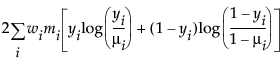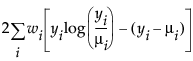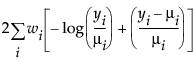An important aspect of generalized linear modeling is the selection of explanatory variables in the model. Changes in goodness-of-fit statistics are often used to evaluate the contribution of subsets of explanatory variables to a particular model. The deviance is defined to be twice the difference between the maximum attainable log-likelihood and the log-likelihood at the maximum likelihood estimates of the regression parameters. The deviance is often used as a measure of goodness of fit. The maximum attainable log-likelihood is achieved with a model that has a parameter for every observation. Deviance Formulas for Response Distributions lists the deviance formula for each of the available distributions for the response variable.
 Distribution Deviance Formula NormalBinomialPoissonExponentialyi is the ith response
μi is the corresponding predicted mean
V(μi) is the variance function
wi is a known weight for the ith observation
Note: If no weight is specified, wi = 1 for all observations.
(yiμi) is the raw residual
sign(yiμi) is 1 if (yiμi) is positive and -1 if (yiμi) is negative
di is the contribution to the total deviance from observation i
φ is the dispersion parameter
V(μi) is the variance function
hi is the ith diagonal element of the matrix We(1/2)X(X'WeX)-1X'We(1/2), where We is the weight matrix used in computing the expected information matrix.

Help created on 9/19/2017